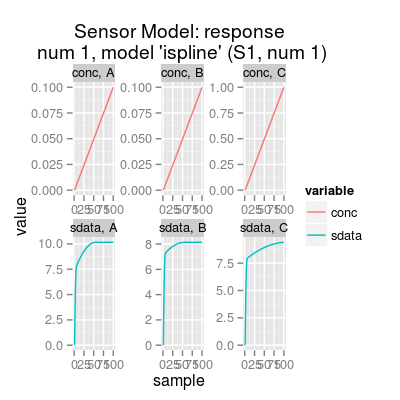# Method coeffNonneg.

## Usage

```SensorModelNames()

defaultParSensorModel()

SensorModel(...)```

## Arguments

...
parameters of constructor.

## Value

Character vector of model names.

List of the default parameters.

## Description

Method coeffNonneg.

Class `SensorModel` predicts a sensor signal in response to an input concentration matrix by means of a regression model stored in slot `dataModel`.

Function to get model names of class `SensorModel`.

Function to get default constructor parameters of class `SensorModel`.

Constructor method of SensorModel Class.

Wrapper function SensorModel.

## Details

The model explicitely assumes that the sensor response to a mixture of analytes is a sum of responses to the individual analyte components. Linear models `mvr` and `plsr` follow this assumtion in their nature.

Slots of the class:

 `num` Sensor number (`1:17`). The default value is `1`. `gases` Gas indices. `ngases` The number of gases. `gnames` Names of gases. `concUnits` Concentration units external to the model, values given in an input concentration matrix. `concUnitsInt` Concentration units internal for the model, values used numerically to build regression models. `dataModel` Data model of class `SensorDataModel` performs a regression (free of the routine on units convertion, etc). `coeffNonneg` Logical whether model coefficients must be non-negative. By default, `FALSE`. `coeffNonnegTransform` Name of transformation to convert negative model coefficients to non-negative values. `beta` (parameter of sensor diversity) A scaling coefficient of how different coefficients of `SensorDataModel` will be in comparision with those coefficients of the UNIMAN sensors. The default value is `2`.

Methods of the class:

 `predict` Predicts a sensor model response to an input concentration matrix. `coef` Extracts the coefficients of a regression model stored in slot `dataModel`.

The `plot` method has two types (parameter `y`):

 `response` (default) Shows the sensitivity curves per gas in normalized concentration units. `predict` Depicts input (parameter `conc`) and ouput of the model for a specified gas (parameter `gases`).

## Examples

```
# sensor model: default initialization
sm <- SensorModel()

# get information about the model
show(sm)

Sensor Model (num 1), beta 2, data model 'ispline'

print(sm)

Sensor Model
- num 1
- beta 2
- 3 gases A, B, C
- (first) data model
- method: ispline (type: spline)
- sensor model: coeffNonneg TRUE
-- coefficients (first): 7.5816, 2.5812, 0 ... 0

print(coef(sm)) # sensitivity coefficients

[,1]
[1,] 7.581598
[2,] 2.581216
[3,] 0.000000
[4,] 7.194504
[5,] 0.946807
[6,] 0.000000
[7,] 7.869399
[8,] 1.551513
[9,] 0.000000

plot(sm)# get available model names
model.names <- SensorModelNames()
print(model.names)

 "plsr"         "mvr"          "broken-stick" "ispline"

# sensor model: custom parameters
sm <- SensorModel(num=7, model="plsr", gases=c(1, 3))

print(sm)

Sensor Model
- num 7
- beta 2
- 2 gases A, C
- (first) data model
- method: plsr (type: mvr)
- ncomp: 2
- sensor model: coeffNonneg FALSE
-- coefficients (first): 0.186, 0.0116

#plot(sm, uniman=TRUE) # add UNIMAN reference data (the model was build from)

# method plot
#  - plot types 'y': response, predict
sm <- SensorModel() # default sensor model

plot(sm, "response", main="plot(sm, 'response')")# default plot type, i.e. 'plot(sm)' does the same plotting

conc <- concSample(sm, "range", gases=1, n=10)
plot(sm, "predict", conc, gases=1, main="plot(sm, 'predict', conc, gases=1)")```

`UNIMANshort`